﻿ Java Polymorphism - Shape Class with Rectangle, Circle and Triangle Subclasses

# Java Polymorphism - Shape Class with Circle, Rectangle, and Triangle Subclasses for Area and Perimeter Calculation

## Java Polymorphism: Exercise-6 with Solution

Write a Java program to create a class Shape with methods getArea() and getPerimeter(). Create three subclasses: Circle, Rectangle, and Triangle. Override the getArea() and getPerimeter() methods in each subclass to calculate and return the area and perimeter of the respective shapes.

In the given exercise, here is a simple diagram illustrating polymorphism implementation: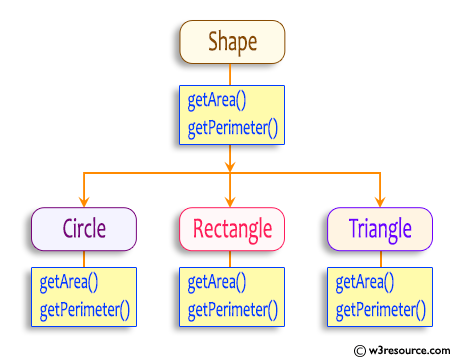In the above diagram, the Circle, Rectangle, and Triangle classes have the getArea() and getPerimeter() methods. These methods allow them to calculate and return the area and perimeter specific to each shape.

Sample Solution:

Java Code:

``````// Shape.java
// Base class Shape
abstract class Shape {
public abstract double getArea();
public abstract double getPerimeter();
}
```
```
``````// Circle.java
// Subclass Circle
class Circle extends Shape {

}

@Override
public double getArea() {
}

@Override
public double getPerimeter() {
return 2 * Math.PI * radius;
}
}
``````
``````// Rectangle.java
// Subclass Rectangle
class Rectangle extends Shape {
private double length;
private double width;

public Rectangle(double length, double width) {
this.length = length;
this.width = width;
}

@Override
public double getArea() {
return length * width;
}

@Override
public double getPerimeter() {
return 2 * (length + width);
}
}
``````
``````// Triangle.java
// Subclass Triangle

class Triangle extends Shape {
private double side1;
private double side2;
private double side3;

public Triangle(double side1, double side2, double side3) {
this.side1 = side1;
this.side2 = side2;
this.side3 = side3;
}

@Override
public double getArea() {
double s = (side1 + side2 + side3) / 2;
return Math.sqrt(s * (s - side1) * (s - side2) * (s - side3));
}

@Override
public double getPerimeter() {
return side1 + side2 + side3;
}
}
``````
``````// Main.java
// Main class
public class Main {
public static void main(String[] args) {
double r = 4.0;
Circle circle = new Circle(r);
double rs1 = 4.0, rs2 = 6.0;
double ts1 = 3.0, ts2 = 4.0, ts3 = 5.0;
Rectangle rectangle = new Rectangle(rs1, rs2);
Triangle triangle = new Triangle(ts1, ts2, ts3);
System.out.println("Area of the Circle: " + circle.getArea());
System.out.println("Perimeter of the Circle: " + circle.getPerimeter());
System.out.println("\nSides of the rectangle are: "+rs1+','+rs2);
System.out.println("Area of the Rectangle: " + rectangle.getArea());
System.out.println("Perimeter of the Rectangle: " + rectangle.getPerimeter());
System.out.println("\nSides of the Traiangel are: "+ts1+','+ts2+','+ts3);
System.out.println("Area of the Triangle: " + triangle.getArea());
System.out.println("Perimeter of the Triangle: " + triangle.getPerimeter());
}
}

``````

Sample Output:

```Radius of the Circle4.0
Area of the Circle: 50.26548245743669
Perimeter of the Circle: 25.132741228718345

Sides of the rectangle are: 4.0,6.0
Area of the Rectangle: 24.0
Perimeter of the Rectangle: 20.0

Sides of the Traiangel are: 3.0,4.0,5.0
Area of the Triangle: 6.0
Perimeter of the Triangle: 12.0
```

Flowchart: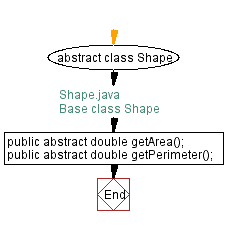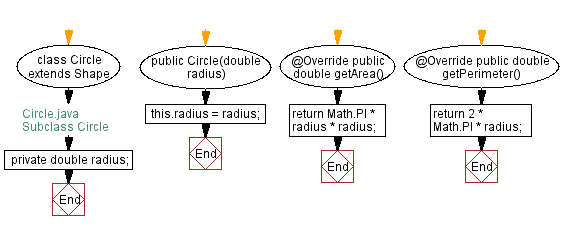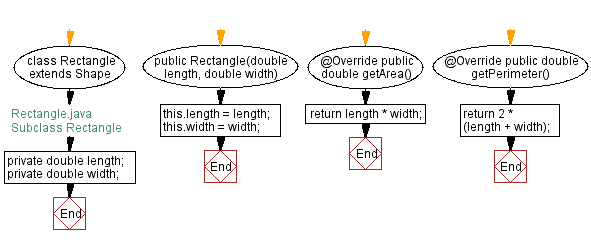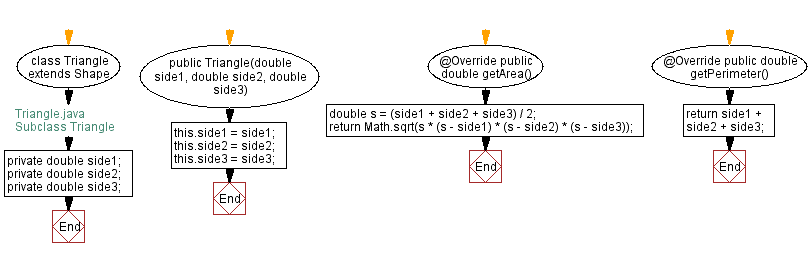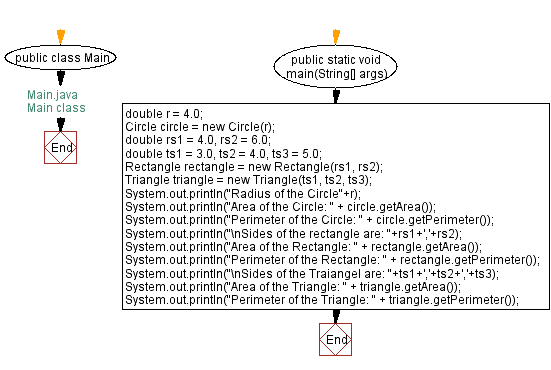Java Code Editor:

What is the difficulty level of this exercise?

﻿

## Java: Tips of the Day

Capturing an exception's stack trace

```import java.io.*;

Exception e = ...;
java.io.StringWriter sw = new java.io.StringWriter();
e.printStackTrace(new java.io.PrintWriter(sw));
String trace = sw.getBuffer().toString();
```

Ref: https://bit.ly/3k8PmGm

We are closing our Disqus commenting system for some maintenanace issues. You may write to us at reach[at]yahoo[dot]com or visit us at Facebook